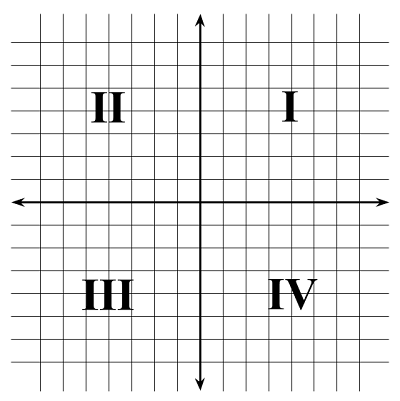# Analytical & Non-Euclidean Geometry Flashcards

Analytical & Non-Euclidean Geometry Flashcards
1/21 (missed) 0 0
Create Your Account To Continue Studying

As a member, you'll also get unlimited access to over 75,000 lessons in math, English, science, history, and more. Plus, get practice tests, quizzes, and personalized coaching to help you succeed.

Try it risk-free for 30 days. Cancel anytime.
###### Already registered? Login here for access
Find the slope, m, of the line given by the equation y = -4x + 3
m = -4
Got it
Find the distance between points A and B if A = (5,-1) and B = (2,3).
The distance between points A and B is 5.
Got it
What is the distance between points A and B if A = (1,0) and B = (-9,-4)?
The distance between points A and B is about 10.8.
Got it
What is the midpoint between (3,9) and (7,-7)?
(5,1)
Got it
What is the midpoint between (-2,3) and (8,1)?
(3,2)
Got it
Which quadrant contains the point (4,-5)?
IV, the fourth quadrant
Got it
Which quadrant has -x & +y values?II, the second quadrant
Got it
Which quadrant has -x & -y values?III, the third quadrant
Got it
Which quadrant has +x & -y values?IV, the fourth quadrant
Got it
Which quadrant has positive x and y values?I, the first quadrant
Got it

### Ready to move on?

or choose a specific lesson: See all lessons in this chapter
21 cards in set

## Flashcard Content Overview

This flashcard set covers the different types of geometries, including hyperbolic geometry, elliptical geometry, and Euclidean geometry. Flashcards in this set will quiz you on the definitions and differences between these types of geometries. This flashcard set also covers basic topics in analytic geometry. Check your understanding of the coordinate plane, quadrants, plotting points and graphing lines, and using the distance and midpoint formulas.

Front
Back
Which quadrant has positive x and y values?I, the first quadrant
Which quadrant has +x & -y values?IV, the fourth quadrant
Which quadrant has -x & -y values?III, the third quadrant
Which quadrant has -x & +y values?II, the second quadrant
Which quadrant contains the point (4,-5)?
IV, the fourth quadrant
What is the midpoint between (-2,3) and (8,1)?
(3,2)
What is the midpoint between (3,9) and (7,-7)?
(5,1)
What is the distance between points A and B if A = (1,0) and B = (-9,-4)?
The distance between points A and B is about 10.8.
Find the distance between points A and B if A = (5,-1) and B = (2,3).
The distance between points A and B is 5.
Find the slope, m, of the line given by the equation y = -4x + 3
m = -4
Find the slope, m, of a line that goes through (2,2) and (8,14).
m = 2
Find the slope, m, of the line 4x - 2y = 2

m = 2

4x - 2y = 2 expressed in slope-intercept form is y = 2x - 1.

Therefore, the slope is 2.

Find the equation of a line passing through the point (2,5) and parallel to the line y = 2x - 8
y = 2x + 1
Parallel postulate

Given a straight line and a point not on that line, there exists only one line that passes through the point and never intersects the original line, regardless of how far the lines are extended.

Euclidean geometry

The geometry of points, lines, and shapes on flat surfaces where the parallel postulate holds true.

Non-Euclidean geometry

The geometry of points, lines, and shapes on curved surfaces where the parallel postulate does not hold true.

Two common types of non-Euclidean geometry

Spherical geometry and hyperbolic geometry

This type of geometry describes saddle shapes.

Hyperbolic geometry

The sum of the interior angles of a triangle will always be equal to 180 degrees in this type of geometry.

Euclidean geometry

The sum of the interior angles of a triangle will always be greater than 180 degrees in this type of geometry.

Spherical geometry

The sum of the interior angles of a triangle will always be less than 180 degrees in this type of geometry.

Hyperbolic geometry

To unlock this flashcard set you must be a Study.com Member.